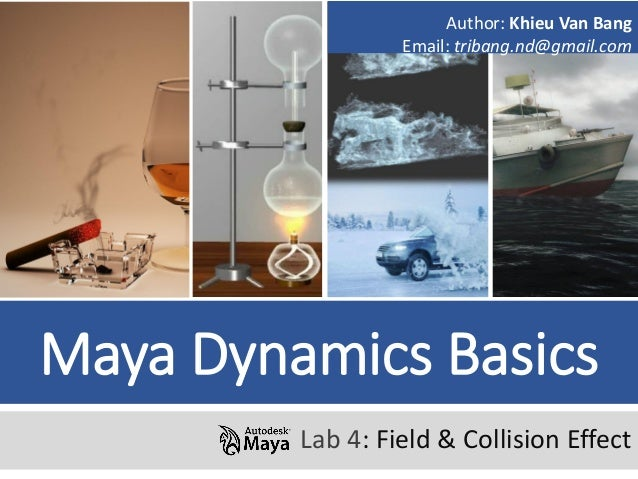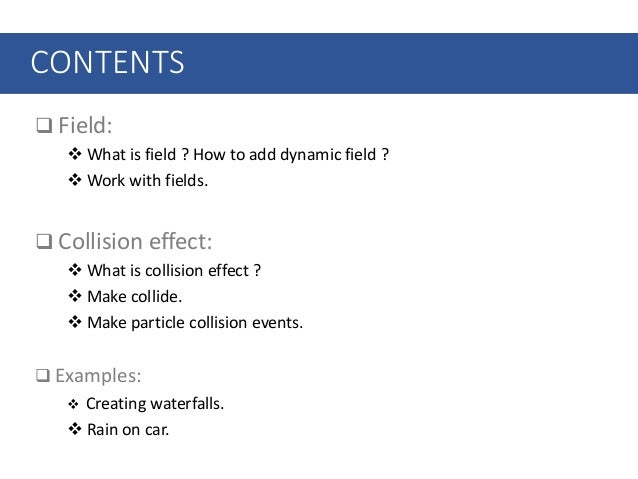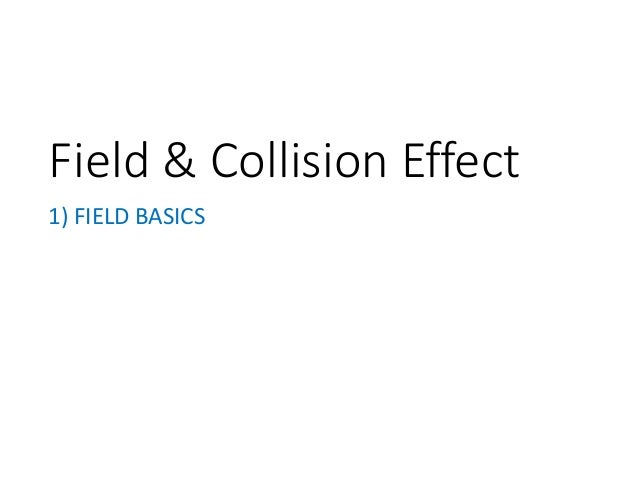Successfully reported this slideshow.Upcoming SlideShare
×

of0

Share

# Session 04 – field &amp; collision effect

1 Field:
 What is field ? How to add dynamic field ?
Work with fields.

2 Collision effect:
 What is collision effect ?
 Make collide.
 Make particle collision events.

3 Examples:
 Creating waterfalls.
 Rain on car.

See all

### Related Audiobooks

#### Free with a 30 day trial from Scribd

See all
• Be the first to like this

### Session 04 – field &amp; collision effect

1. 1. Maya Dynamics Basics Lab 4: Field & Collision Effect Author: Khieu Van Bang Email: tribang.nd@gmail.com
2. 2. CONTENTS  Field:  What is field ? How to add dynamic field ?  Work with fields.  Collision effect:  What is collision effect ?  Make collide.  Make particle collision events.  Examples:  Creating waterfalls.  Rain on car.
3. 3. Field & Collision Effect 1) FIELD BASICS
4. 4. 1) FIELD BASICS 1.1) What is field ? How to add dynamic field ? 1.2) Work with fields. Field & Collision Effect
5. 5. 1.1) What is Field ? 1) FIELD BASICS Fields You can simulate the motion of natural forces with dynamic fields. For example, you can connect a vortex field to emitted particles to create swirling motion. Types of fields  Air fields.  Drag fields.  Gravity fields.  Newton fields.  Radial fields.  Turbulence fields.  Uniform fields.  Vortex fields.  Volume Axis fileds.
6. 6. 1) FIELD BASICS Fields - Air o An air field simulates the effects of moving air. The objects you connect to the air field accelerate or decelerate so their velocities match that of the air as the animation plays. o You can parent an air field to a moving part of an object to simulate a wake of air from the moving part. Types of Field
7. 7. 1) FIELD BASICS Fields - Air Types of Field o Wind: effects of wind. o Wake: movement of air disrupted and pulled along by a moving object. o Fan: a local fan effect. o Magnitude: strength of the air field. o Attenuation: strength of the field diminishes as distance to the affected object increases. o Direction X, Y, Z :Specifies the direction the air blows. o Speed: Controls how quickly the connected objects match the velocity of the air field. o Inherit Rotation: rotating or parented to a rotating object, the air flow undergoes that same rotation. o Component Only: direction specified by the combination of its Direction, Speed, and Inherit Velocity attributes. o Enable Spread: Specifies whether to use the Spread angle. o Spread : Represents the angle from the Direction settings within which objects are affected by the air field. o Use Max Distance: connected objects within the area defined by the Max Distance. o Max Distance: Sets the maximum distance from the air field at which the field is exerted.
8. 8. 1) FIELD BASICS Types of Field Fields - Drag o A drag field exerts a friction or braking force on an object that’s animated with dynamic motion.
9. 9. 1) FIELD BASICS Types of Field Fields - Drag o Magnitude: Sets the strength of the drag field. The greater the magnitude, the greater the braking force on the moving object. o Attenuation: Sets how much the strength of the field diminishes as distance to the affected object increases. The rate of change is exponential with distance; the Attenuation is the exponent. If you set Attenuation to 0, the force remains constant over distance. o Use Direction: Specifies that the braking force is exerted only against the object’s velocity that lies along Direction X, Y, Z. Available in the Attribute Editor only. o Use Max Distance: If you turn on Use Max Distance, connected objects within the area defined by the Max Distance setting are affected by the drag field. Any connected objects outside the Max Distance are not affected by the drag field. Available in Attribute Editor only.
10. 10. 1) FIELD BASICS Types of Field Fields - Gravity o A gravity field simulates the Earth's gravitational force. It accelerates objects in a fixed direction.
11. 11. 1) FIELD BASICS Types of Field Fields - Gravity o Magnitude: Sets the strength of the gravity field. The greater the magnitude, the faster the objects will accelerate in the direction of the gravitational force. o Attenuation: Sets how much the strength of the field diminishes as distance to the affected object increases. The rate of change is exponential with distance; the Attenuation is the exponent. If you set Attenuation to 0, the force remains constant over distance. o Direction X, Y, Z: Sets the direction of the gravitational force. o Use Max Distance: If you turn on Use Max Distance, connected objects within the area defined by the Max Distance setting are affected by the gravity field. Any connected objects outside the Max Distance are not affected by the gravity field.
12. 12. 1) FIELD BASICS Types of Field Fields - Newton o A newton field pulls objects towards it. This lets you create effects such as orbiting planets or tethered, colliding balls. This field is based on the principle that a mutual attractive force exists between any two objects in the universe, proportional to the product of their masses. As the distance between the objects increases, the force of the pull decreases.
13. 13. 1) FIELD BASICS Types of Field Fields - Newton o Magnitude: strength of the newton field o Attenuation : strength of the field diminishes as distance to the affected object increases. o Min Distance: minimum distance from the newton field. o Use Max Distance: connected objects within the area defined. o Max Distance: maximum distance from the newton field.
14. 14. 1) FIELD BASICS Types of Field Fields - Radial o A radial field pushes objects away or pulls them toward itself, like a magnet.
15. 15. 1) FIELD BASICS Types of Field Fields - Radial o Magnitude: strength of the radial field. o Attenuation: strength of the field diminishes as distance to the affected object increases. o Radial Type: Specifies how the radial field’s effect diminishes with Attenuation. o Use Max Distance : connected objects. o Max Distance : maximum distance from the radial field that the field is exerted.
16. 16. 1) FIELD BASICS Types of Field Fields - Turbulence Soft body before being connected to turbulence field. Soft body after being connected to turbulence field. o A turbulence field causes irregularities in the motion of affected objects. These irregularities are also called noise or jitter. You can combine turbulence with other fields to mimic the random motion in fluid or gaseous mediums such as water and air.
17. 17. 1) FIELD BASICS Types of Field Fields - Turbulence o Frequency: frequency of the turbulence field. o Phase X, Y, Z : phase shift of the turbulence field. o Interpolation Type : Linear specifies a linear interpolation between values in the noise table. o Noise Level: The greater the value, the more irregular the turbulence. o Noise Ratio: Specifies the weighting of successive lookups. The weights are cumulative.
18. 18. 1) FIELD BASICS Types of Field Fields - Uniform o A uniform field pushes objects in a uniform direction.
19. 19. 1) FIELD BASICS Types of Field Fields - Uniform o Magnitude : strength of the uniform field. o Attenuation : strength of the field diminishes as distance to the affected object increases. o Direction X, Y, Z : Specifies the direction the uniform field pushes objects. o Use Max Distance : connected objects within the area defined by the Max Distance setting are affected by the uniform field. o Max Distance : maximum distance from the uniform field that the field is exerted.
20. 20. 1) FIELD BASICS Types of Field Fields - Vortex o A vortex field pulls objects in a circular or spiraling direction. You can use this field with particles to create effects such as whirlpools or tornados.
21. 21. 1) FIELD BASICS Types of Field Fields - Vortex o Magnitude : strength of the vortex field. o Attenuation : strength of the field diminishes as distance to the affected object increases. o Axis X, Y, Z : Specifies the axis around which the vortex field exerts its force. o Use Max Distance : connected objects within the area defined by the Max Distance setting are affected by the vortex field. o Max Distance : maximum distance from the vortex field that the field is exerted.
22. 22. 1) FIELD BASICS Types of Field Fields – Volume axis  The Volume Axis field lets you move objects, including particles and nParticles, in various directions in a volume. The motion imparted on the object is relative to the axis of the volume.  You can use the Volume Axis field to create effects such as particles flowing around obstacles, solar flares, mushroom clouds, explosions, tornadoes, and rocket exhaust.
23. 23. 1) FIELD BASICS Types of Field Fields – Volume axis o Magnitude : Specifies the strength of the volume axis field. o Attenuation: strength of the field diminishes from the central axis of the volume axis field. o Max Distance : Sets the maximum distance at which the field has an effect. o Volume Shape : Specifies the closed volume that defines the area in which the volume axis field affects particles and rigid bodies. o Volume Offset X, Y, Z: Offsets the volume axis field from the actual location of the field. o Volume Sweep : Defines the extent of the rotation for all volume shapes except cube. This can be a value from 0 to 360 degrees. o Section Radius : Defines the thickness of the solid portion of a torus volume shape. o Invert Attenuation : strength of the volume axis field is strongest at the edge.
24. 24. 1) FIELD BASICS Types of Field Fields – Volume axis o Away From Center : move away from the center point of cube or sphere volumes. o Away From Axis : move away from the central axis of cylinder, cone, or torus volumes. o Along Axis : move along the central axis of all volumes. o Around Axis : move around the central axis of all volumes. o Directional Speed : Adds speed in the direction specified. o Direction X, Y, Z : Moves particles in the directions specified by the X, Y, and Z axes. o Turbulence : intensity of a force simulating a turbulent wind that evolves over time. o Turbulence Speed : rate of change of the turbulence over time. o Turbulence Frequency X, Y, Z : repeats of the turbulence function fit inside the bounding volume of the emitter. o Turbulence Offset X, Y, Z : translate the turbulence within the volume. Animating it can simulate blowing turbulent wind. o Detail Turbulence : relative intensity of a second higher frequency turbulence.
25. 25. 1) FIELD BASICS 1.1) What is field ? How to add dynamic field ? 1.2) Work with fields. Field & Collision Effect
26. 26. 1) FIELD BASICS 1.2) Work with fields  Create fields 1
27. 27.  Connect objects to the field 1) FIELD BASICS 1.2) Work with fields o Use Dynamic Relationships 1 2
28. 28.  Edit field attributes 1) FIELD BASICS 1.2) Work with fields Step 1: Select the field you want to edit. You can select the particle object connected to the field and the Attribute Editor displays a tab for the field. Step 2: Do one of the following: o Use the Attribute Editor or Channel Box to set the attributes. The attributes are described in the field descriptions in this chapter. o Use the workspace manipulators to edit commonly used field attributes.
29. 29. Field & Collision Effect 2) COLLISSION EFFECT
30. 30. Field & Collision Effect 2) COLLISION EFFECT 2.1) What is collision effect ? 2.2) Make collide. 2.3) Edit connector. 2.4) Make particle collision events.
31. 31. 2.1) What is collision effect ? 2) COLLISION EFFECT Collision effect is an event effect in which two or more bodies exert forces on each other for a relatively short time.
32. 32. Field & Collision Effect 2) COLLISION EFFECT 2.1) What is collision effect ? 2.2) Make collide. 2.3) Edit connector. 2.4) Make particle collision events.
33. 33. 2) COLLISION EFFECT 2.2) Make collide 1 2 3  What is collide ?  How to create a collider?
34. 34. 2) COLLISION EFFECT 2.2) Make collide How to create it ?
35. 35. Field & Collision Effect 2) COLLISION EFFECT 2.1) What is collision effect ? 2.2) Make collide. 2.3) Edit connector. 2.4) Make particle collision events.
36. 36.  Tesselation Fator: attribute represents the approximate number of polygons in the tessellated surface.  Reslience: sets how much the colliding particle’s velocity parallel to the surface decreases or increases as it bounces off the collision surface. 2) COLLISION EFFECT 2.3) Edit Connector  Friction: rebound occurs. A value of 0 makes particles collide with no bounce. A value of 1 causes particles to rebound fully.  Offeset.
37. 37. 2) COLLISION EFFECT 2.3) Edit Connector  Tesselation Fator
38. 38. 2) COLLISION EFFECT 2.3) Edit Connector  Reslience
39. 39. 2) COLLISION EFFECT 2.3) Edit Connector  Friction
40. 40. Field & Collision Effect 2) COLLISION EFFECT 2.1) What is collision effect ? 2.2) Make collide. 2.3) Edit connector. 2.4) Make particle collision events.
41. 41. 2) COLLISION EFFECT 2.4) Make particle collision events.  Event effect in which two or more bodies exert forces on each other for a relatively short time. Object 1 Object 2
42. 42. 2) COLLISION EFFECT 2.4) Make particle collision events. 4 5 6 7 8  Create a collision event in Maya. 2 1 3
43. 43. Field & Collision Effect 3) EXAMPLES
44. 44. 3.1) Creating waterfalls. 3.2) Rain splashing on the floor. Field & Collision Effect 3) EXAMPLES
45. 45. 3) EXAMPLES 3.1) Creating star effect. Using Turbulence field.
46. 46. 3.1) Water poured into a glass. 3.2) Rain on car. Field & Collision Effect 3) EXAMPLES
47. 47. 3) EXAMPLES 3.2) Rain on car. This example is a form of Collision effect.
48. 48. 3) EXAMPLES You can create it
49. 49. Thanks!

Total views

288

On Slideshare

0

From embeds

0

Number of embeds

4

16

Shares

0